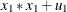### NLINCON Statement

`NLINCON | NLC` constraint <, constraint …> ;

where constraint represents one of the following:number operator variable-list number operatorvariable-list number operatornumber operator variable-list

You can specify nonlinear equality and inequality constraints with the NLINCON or NLC statement. The QUANEW optimization subroutine is used when you specify nonlinear constraints by using the NLINCON statement.

The syntax of the NLINCON statement is similar to that of the BOUNDS statement, except that the NLINCON statement must contain the names of variables that are defined in the program statements and are defined as continuous functions of parameters in the model. They must not be confused with the variables in the data set.

As with the BOUNDS statement, one- or two-sided constraints are allowed in the NLINCON statement; equality constraints must be one sided. Valid operators are <=, <, >=, >, and = (or, equivalently, LE, LT, GE, GT, and EQ).

PROC CALIS cannot enforce the strict inequalities < or > but instead treats them as <= and >=, respectively. The listed nonlinear constraints must be separated by commas. The following is an example of the NLINCON statement that constrains the nonlinear parametric functionto a fixed value of 1:

```nlincon    xx = 1;
xx = x1 * x1 + u1;
```

Note that `x1` and `u1` are parameters defined in the model. The following three NLINCON statements, which require `xx1`, `xx2`, and `xx3` to be between zero and ten, are equivalent:

```nlincon  0. <= xx1-xx3,
xx1-xx3 <= 10;
nlincon 0. <= xx1-xx3 <= 10.;
nlincon 10. >= xx1-xx3 >= 0.;
```